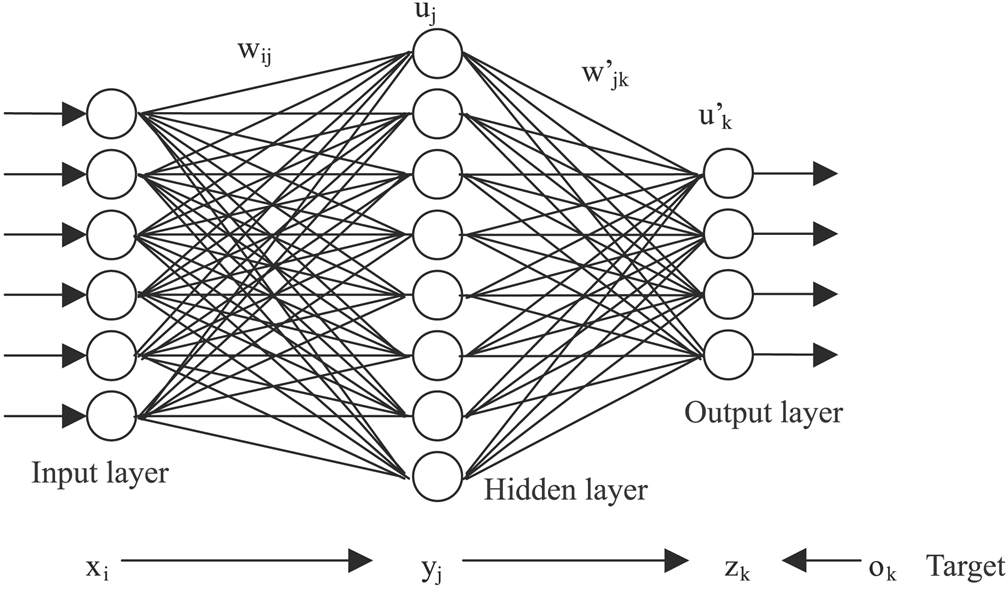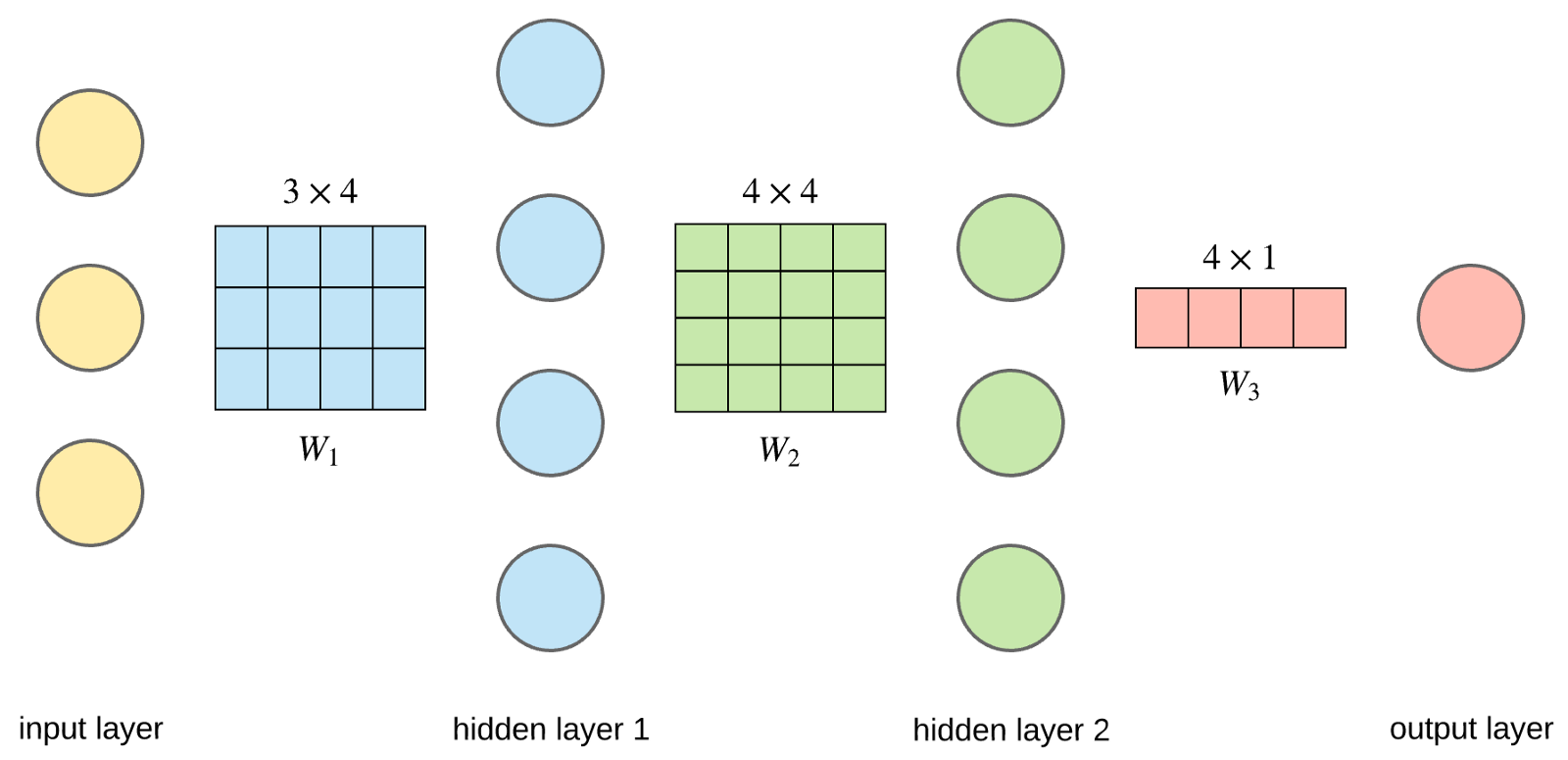# Building a Neural Network Library From Scratch with Python## Why?

This blog post is intended to act as an introduction to a future post which will cover neuroevolution. The reasons why I made a neural network library from scratch was that I found it difficult to extract the weights of each neuron after training in TensorFlow and that I didn’t really need a complex library, I just needed a simple neural network that can take an input and provide an output, heck, I didn’t even need backpropagation.

## What Kind of Neural Network is it?

The image above is exactly what I’m going to be building, a three-layer, fully connected neural network with one input layer, one hidden layer, and one output layer. I offer flexibility in the number of neurons in each layer, and you can extract the weights of each neuron and modify it however you wish to do so.

## How Does a Neural Network Work?

Let’s us consider the linear equation

`Y = mX+ b`

`X` is the input for the equation, `m` is the weight, and `b` is the bias. Each neuron in a simple neural network does this exact thing. Takes the input that is given, multiplies it with a weight and adds a bias. Now when it comes to neural networks, a neuron can have more than one input(like the image above) and so each input connection will it’s own input weight which is used to multiply the value coming from that connection. All these multiplied values from each connection are then added up and the bias is added. This value goes through an activation function and is given as an output.

Activation functions come in different varieties but they all essentially have the same purpose. Let us consider that a movie is rated 70/100. We, humans, know that it is essentially the same thing as 7/10 and if we were asked to do some numeric operations (multiplying big numbers, adding big numbers) on 70/100, we would naturally reduce it to a simpler form (7/10) and then carry out the operations. Just like us, it’s easier(takes less space, and marginally faster) for a computer to do operations on smaller numbers. Essentially, activations functions serve the purpose of converting larger space to a smaller space. 70/100 is on a space that ranged from 0 to 100, but 7/10 is a space that’s a tenth of the size, ranging from 0 to 10.

## A Little Theory Before Coding

Before we start coding, I would like to explain the 3 steps that happen when data flows through a neural network

#### 1. Weight Multiplication

The weights of a neural network can be represented in a matrix. Assume there are two layers, the input layer and the hidden layer and we want to represent these weights in a matrix. Also let us assume that there are 3 input nodes and 4 hidden nodes. Since this neural network is going to be a fully connected one, there are going to be 12 weight values totally.

hidden1 hidden2 hidden3 hidden4
input1 val1 val2 val3 val4
input2 val5 val6 val7 val8
input3 val9 val10 val11 val12Another benefit of representing weights in a matrix form is that, if we form a matrix out of the inputs, we jsut have to do matrix multiplication of these two and we get the output of the hidden layer.

Let’s assume there are 3 input nodes, so we will have a 3x1 matrix. There are 4 hidden nodes so the weight matrix between the input and the output layers will be a 3x4 matrix.

If we multiply `transpose(inputMatrix) x weightMatrix` we will get a 1x4 matrix. These are the values that go into each of the hidden layer nodes after the weight is multiplied to each input and added up. You can see now how representing everything as matrices helps speed up the calculation as multiplication of the weights and the summing up of all the values is done in one single operation.

All the layers apart from the input layer have biases. These are simple 1xn matrices that hold the bias values. After the weight multiplication step is done, the bias matrix is added to it. This bias matrix can be thought of as the `b` in a linear equation.

#### 3. Activation

Now that we have an array (1xn) matrix we need to map the values to a smaller domain. Any activation function can be used to reduce each of the values in this matrix.

## Code

Let us encapsulate our neural network code into a class. So we can easily use that to do the initialization, predicting output and all that.

Structure

``````import numpy as np
class NeuralNetwork():

def __init__(self, numInput, numHidden, numOutput):
pass

def predict(self, input):
pass
``````

As I mentioned before this is going to be a three layer neural network. So our class will require three inputs

• `numInput`: the number of input layer nodes
• `numHidden`: the number of hidden layer nodes
• `numOutput`: the number of output nodes

The `__init__` function to initialize the weights randomly and create the approriate sized matrices for further calculation.

Initialization

``````def __init__()(self, numInput, numHidden, numOutput):
self.inputHiddenWeights = np.random.normal(size=(numInput, numHidden))
self.hiddenOutputWeights = np.random.normal(size=(numHidden, numOutput))

self.hiddenBias = np.random.normal(size=(numHidden, 1))
self.outputBias = np.random.normal(size=(numOutput, 1))
``````

Now let’s fill the code for propagating the input data all the way to the output layer. This step is also called forward propagation.

Forward Propagation

``````def predict(self, input):
# Weight Multiplication
self.hidden = np.dot(np.array(input).T, self.inputHiddenWeights).reshape(-1, 1)
# Activation
self.hidden = self.sigmoid(self.hidden)

# Weight Multiplication
self.output = np.dot(self.hidden.T, self.hiddenOutputWeights).reshape((-1, 1))
# Activation
self.output = self.sigmoid(self.output)
``````
• `.reshape(-1, 1)` converts a matrix into an nx1 matrix
• `.reshape(-1)` converts a matrix into a 1xn matrix
• `.dot()` does matrix multiplication of the two inputs

There is one problem though, we haven’t defined the sigmoid activation function.

Sigmoid Activation

``````def sigmoid(input):
return 1 / (1 + np.exp(-x))
``````

Putting Everything Together

``````import numpy as np
class NeuralNetwork():

def __init__(self, numInput, numHidden, numOutput):
self.inputHiddenWeights = np.random.normal(size=(numInput, numHidden))
self.hiddenOutputWeights = np.random.normal(size=(numHidden, numOutput))

self.hiddenBias = np.random.normal(size=(numHidden, 1))
self.outputBias = np.random.normal(size=(numOutput, 1))

def predict(self, input):
# Weight Multiplication
self.hidden = np.dot(np.array(input).T, self.inputHiddenWeights).reshape(-1, 1)
# Activation
self.hidden = self.sigmoid(self.hidden)

# Weight Multiplication
self.output = np.dot(self.hidden.T, self.hiddenOutputWeights).reshape((-1, 1))